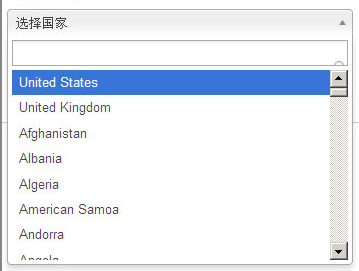# jQuery Ajax实现Select多级关联动态绑定数据的实例代码

更新时间：2018年10月26日 10:11:15   作者：技术小能手我要评论

jQuery选择插件分为基本版和美化版，重点说下美化版，如下图所示：jsp页面：

```<select class=”chzn-select” id="CODE" name="CODE">
......
</select>```

js页面：

`\$(".chzn-select").chosen();`

```<!-- 省份 -->
<select class="chzn-select" id="ShengFen" name="ShengFen" onChange="setCity();" data-placeholder="请选择省份">
<option value=""></option>
<option value="">全部</option>
<c:foreach items="\${sfList}" var="sf">
<option value="\${sf.SF_ID}" <c:if test="\${pd.sfId == sf.SF_ID}">selected</c:if>>\${sf.SF_NAME}
</option>
</c:foreach>
</select>
<!-- 城市 -->
<select class="chzn-select" id="City" name="City" data-placeholder="请选择城市">
<option value=""></option>
<option value="">全部</option>
<c:foreach items="\${cyList}" var="cy">
<option value="\${cy.CY_ID}" <c:if test="\${pd.cyId == cy.CY_ID}">selected</c:if>>\${cy.CY_NAME}
</option>
</c:foreach>
</select>```

```//下拉框动态关联
function setCity(){
// 获取选择的省份的ID
var sfId = \$("#ShengFen").val();
\$.ajax({
type : "POST",
url : "SFAndCity/setCity.do",
data : {"sfId":sfId},
dataType : "json",
success : function(data){
var cyList = data.cyList;
// 移除以前的绑定数据
\$("#City option").remove();
// 美化一下select（可以去除）
var _option = "<option value=\"\"";
_option += "></option>";
_option += "<option value=\"\"";
_option += ">全部</option>";
// 绑定数据
if(cfList && cyList.length != 0){
\$("select[name=City]").append(_option);
for(var i=0;i<cyList.length;i++){
var option = "<option value=\""+cyList.CY_ID+"\"";
option += ">"+cyList.CY_NAME+"</option>";
\$("select[name=City]").append(option);
}
}else{
\$("select[name=City]").append(_option);
}
// 保证jQuery的选择插件动态绑定数据生效
\$("#City").trigger("liszt:updated");
\$("#City").chosen();
}
});
}```

```@RequestMapping(value="/setCity")
@ResponseBody
public Map<String,Object> setCity(){
Map<String,Object> map = new HashMap<String,Object>();
PageData pd = new PageData();
try{
pd = this.getPageData();
String sfId = pd.getString("sfId"); //和ajax中传递的保持一致
pd.put("CY_SFID",sfId);
List<PageData> cyList = cyService.listCityBysfId(pd);
map.put("cyList",cyList);  //和ajax中获取的保持一致
return map;
}catch(Exception e){

}
return map;
}```